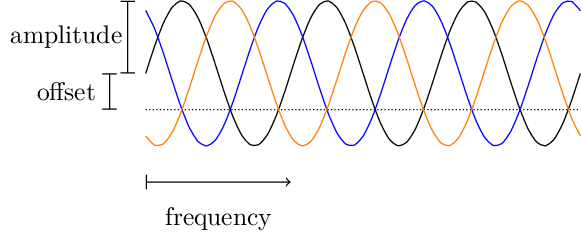# Three phase sine function

uz_3ph_abc_t uz_wavegen_three_phase_sample(float amplitude, float frequency_Hz, float offset)

Samples the global timer and returns a three phase sine wave.

Parameters
• amplitude – Amplitude of all three signals

• frequency_Hz – Frequency in Hertz

• offset – Offset

Returns

uz_3ph_abc_t

## Example

The current value of the three phases at the current system time is calculated by calling `uz_wavegen_three_phase_sample()`. The module uses the `uz_3ph_abc_t` data type from Coordinate Transformation to return all three phase values as a struct.

```float amplitude = 2.0f;
float frequency = 5.0f;
float offset = 1.0f;
uz_3ph_abc_t three_phase_sine = uz_wavegen_three_phase_sample(amplitude, frequency, offset);
```

## Description

Outputs one sample of a sine wave with three phases for each function call.Fig. 73 three phase sine wave